﻿ The Chaotic Monopoly Profit Growth Model and a Pigouvian Tax

The Chaotic Monopoly Profit Growth Model and a Pigouvian Tax

Vesna D. JablanovicOPEN ACCESSPEER-REVIEWED

The Chaotic Monopoly Profit Growth Model and a Pigouvian Tax

Vesna D. JablanovicAbstract

Deterministic chaos refers to irregular or chaotic motion that is generated by nonlinear systems. The chaotic behavior is not to quantum-mechanical-like uncertainty. Chaos theory is used to prove that erratic and chaotic fluctuations can indeed arise in completely deterministic models. Chaotic systems exhibit a sensitive dependence on initial conditions. Seemingly insignificant changes in the initial conditions produce large differences in outcomes. The basic aim of this paper is to construct a relatively simple chaotic growth model of the monopoly price that is capable of generating stable equilibria, cycles, or chaos. A key hypothesis of this work is based on the idea that the coefficient μ = f (n - b - d ) plays a crucial role in explaining local stability of the monopoly profit, where, b - the coefficient of the total cost function of the monopoly firm, n - the coefficient of the inverse demand function, d - the Pigovian tax rate.μ

At a glance: Figures

1
Prev Next

• Jablanovic, Vesna D.. "The Chaotic Monopoly Profit Growth Model and a Pigouvian Tax." Journal of Business and Management Sciences 1.1 (2013): 1-3.
• Jablanovic, V. D. (2013). The Chaotic Monopoly Profit Growth Model and a Pigouvian Tax. Journal of Business and Management Sciences, 1(1), 1-3.
• Jablanovic, Vesna D.. "The Chaotic Monopoly Profit Growth Model and a Pigouvian Tax." Journal of Business and Management Sciences 1, no. 1 (2013): 1-3.

 Import into BibTeX Import into EndNote Import into RefMan Import into RefWorks

1. Introduction

Chaos theory attempts to reveal structure in unpredictable dynamic systems. It is important to construct deterministic, nonlinear economic dynamic models that elucidate irregular, unpredictable economic behavior. Deterministic chaos refers to irregular or chaotic motion that is generated by nonlinear systems evolving according to dynamical laws that uniquely determine the state of the system at all times from a knowledge of the system's previous history. Chaos embodies three important principles: (i) extreme sensitivity to initial conditions; (ii) cause and effect are not proportional; and (iii) nonlinearity.

Chaos theory can explain effectively unpredictable economic long time behavior arising in a deterministic dynamical system because of sensitivity to initial conditions. A deterministic dynamical system is perfectly predictable given perfect knowledge of the initial condition, and is in practice always predictable in the short term. The key to long-term unpredictability is a property known as sensitivity to (or sensitive dependence on) initial conditions.

Chaos theory started with Lorenz's  discovery of complex dynamics arising from three nonlinear differential equations leading to turbulence in the weather system. Li and Yorke  discovered that the simple logistic curve can exibit very complex behavior. Further, May  described chaos in population biology. Chaos theory has been applied in economics by Benhabib and Day [1, 2], Day [3, 4], Grandmont , Goodwin , Medio [15, 16], Lorenz , Jablanovic [7, 8, 9, 10], among many others.

The basic aim of this paper is to provide a relatively simple chaotic the monopoly price growth model that is capable of generating stable equilibria, cycles, or chaos.

2. A Simple Chaotic Price Growth Model of a Profit-Maximizing Monopoly

In the model of a profit-maximizing monopoly, take the inverse demand function(1)

Where P- monopoly price; Q – monopoly output; n, m – coefficients of the inverse demand function.

In this case, total revenue is given by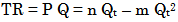(2)

Further, suppose the quadratic total-cost function for a monopoly is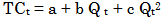(3)

TC – marginal cost; Q – monopoly output ; a, b, c – coefficients of the quadratic marginal-cost function.

It is supposed that the Pigovian tax is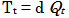(4)

T - the Pigovian tax , Q – monopoly output ; d - the Pigovian tax rate

In this sense , the total cost function for monopoly is(5)

TC –total cost; Q –monopoly output; a, b, c –coefficients of the quadratic total cost function, d - the Pigovian tax rate Profit, Π, is the difference between total revenue and total cost. It is supposed that a=0. Then,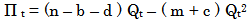(6)

Further , it is supposed that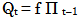(7)

where: Q –monopoly output, Π- monopoly profit.

By substitution one derives:(8)

Further, it is assumed that the monopoly profit is restricted by its maximal value in its time series. This premise requires a modification of the growth law. Now, the monopoly profit growth rate depends on the current size of the monopoly profit, Π, relative to its maximal size in its time series Π m. We introduce π as π = Π / Π m. Thus π range between 0 and 1. Again we index π by t, i.e., write π t to refer to the size at time steps t = 0,1,2,3,... Now, growth rate of the monopoly profit is measured as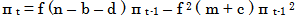(9)

This model given by equation (9) is called the logistic model. For most choices of b, c, d, m, and n there is no explicit solution for (9). Namely, knowing b, c, d, m, and n and measuring π 0 would not suffice to predict πt for any point in time, as was previously possible. This is at the heart of the presence of chaos in deterministic feedback processes. Lorenz  discovered this effect - the lack of predictability in deterministic systems. Sensitive dependence on initial conditions is one of the central ingredients of what is called deterministic chaos.

This kind of difference equation (9) can lead to very interesting dynamic behavior, such as cycles that repeat themselves every two or more periods, and even chaos, in which there is no apparent regularity in the behavior of π t. This difference equation (9) will possess a chaotic region. Two properties of the chaotic solution are important: firstly, given a starting point π 0 the solution is highly sensitive to variations of the parameters b, c, d, m, and n; secondly, given the parameters b, c, d, m, and n the solution is highly sensitive to variations of the initial point π 0. In both cases the two solutions are for the first few periods rather close to each other, but later on they behave in a chaotic manner.

3. Logistic Equation

The logistic map is often cited as an example of how complex, chaotic behavior can arise from very simple non-linear dynamical equations. The logistic model was originally introduced as a demographic model by Pierre François Verhulst. It is possible to show that iteration process for the logistic equation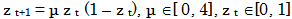(10)

is equivalent to the iteration of growth model (9) when we use the following identification:(11)

Using (9) and (11) we obtainOn the other hand, using (10), (11), and (12) we obtainThus we have that iteratingis really the same as iteratingusingsee Figure 1.

It is important because the dynamic properties of the logistic equation (10) have been widely analyzed [11, 14].

It is obtained that

(i) For parameter values 0 < μ < 1 all solutions will converge to z = 0;

(ii) For 1 < μ < 3,57 there exist fixed points the number of which depends on μ;

(iii) For 1 < μ < 2 all solutions monotnically increase to z = (μ - 1 ) / μ;

(iv) For 2 < μ < 3 fluctuations will converge to z = (μ - 1 ) / μ;

(v) For 3 < μ < 4 all solutions will continously fluctuate;

(vi) For 3,57 < μ < 4 the solution become "chaotic" wihch means that there exist totally aperiodic solution or periodic solutions with a very large, complicated period. This means that the path of zt fluctuates in an apparently random fashion over time, not settling down into any regular pattern whatsoever.

Figure 1. Two quadratic iteratiors running in phase are tightly coupled by the transformations indicated

4. Conclusion

This paper suggests conclusion for the use of the simple chaotic model of a profit -maximizing monopoly in predicting the fluctuations of the monopoly price. The model (9) has to rely on specified parameters parameters b, c, d, m, and n , and initial value of the monopoly profit, π0. But even slight deviations from the values of parameters parameters b, c, d, m, and n and initial value of the monopoly profit, π0., show the difficulty of predicting a long-term behavior of the monopoly profit.

A key hypothesis of this work is based on the idea that the coefficient μ =f (n - b - d ) plays a crucial role in explaining local stability of the monopoly profit , where, b - the coefficient of the total cost function of the monopoly firm, n - the coefficient of the inverse demand function, d - the Pigovian tax rate.

References

  Benhabib, J., & Day, R. H. Rational choice and erratic behaviour. Review of Economic Studies, 48, 459-471. 1981.In article CrossRef  Benhabib, J., & Day, R. H. Characterization of erratic dynamics in the overlapping generation model. Journal of Economic Dynamics and Control, 4, 37-55. 1982.In article CrossRef  Day, R. H. Irregular growth cycles. American Economic Review, 72, 406-414.1982.In article  Day, R. H. The emergence of chaos from classica economic growth. Quarterly Journal of Economics, 98, 200-213.1983.In article  Goodwin, R. M Chaotic economic dynamics. Oxford: Clarendon Press.1990.In article CrossRef  Grandmont, J. M. On enodgenous competitive business cycles. Econometrica, 53, 994-1045.1985.In article  Jablanović, V. Budget Deficit and Chaotic Economic Growth Models. Aracne editrice.S.r.l. 2012a.In article  Jablanović, V. The Chaotic General Economic Equilibrium Model and Monopoly Asian Journal of Business Management 4(4): 373-375, 2012b.In article  Jablanović, V. The Lerner Index and the Chaotic Monopoly Output Growth Model Asian Journal of Business and Management Sciences (AJBMS), 1(12): 102-106, 2012c.In article  Jablanović, V.. The Chaotic Cost-Plus Pricing Model, Australian Journal of Business and Management Research (AJBMR), 2(1): 46-50, 2012d.In article  Li, T., & Yorke, J. Period three implies chaos. American Mathematical Monthly, 8, 985-992, 1975.In article CrossRef  Lorenz, E. N. Deterministic nonperiodic flow. Journal of Atmospheric Sciences, 20, 130-141, 1963.In article CrossRef  Lorenz, H. W.Nonlinear dynamical economics and chaotic motion (2nd ed.). Heidelberg: Springer-Verlag.1993.In article CrossRef  May, R. M. Mathematical models with very complicated dynamics. Nature, 261, 459-467, 1976.In article CrossRef PubMed  Medio, A. Chaotic dynamics: Theory and applications to economics. Cambridge: Cambridge University Press.1993.In article  Medio, A..Chaotic dynamics. Theory and applications to economics, Cambridge University Press, In De Economist, 144(4), 695-698, 1996.In article  Salvatore D. Microeconomics. Addison -Wesley, 1997.In article PubMed